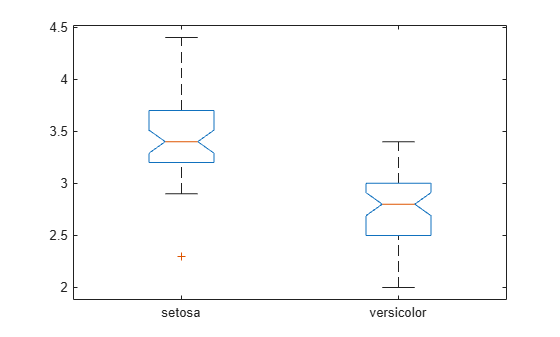Documentation

## Box Plots

Box plots provide a visualization of summary statistics for sample data and contain the following features:

• The tops and bottoms of each “box” are the 25th and 75th percentiles of the samples, respectively. The distances between the tops and bottoms are the interquartile ranges. You can compute the value of the interquartile range using `iqr`.

• The line in the middle of each box is the sample median. If the median is not centered in the box, it shows sample skewness. You can compute the value of the median using the `median` function.

• The whiskers are lines extending above and below each box. Whiskers are drawn from the ends of the interquartile ranges to the furthest observations within the whisker length (the adjacent values).

• Observations beyond the whisker length are marked as outliers. By default, an outlier is a value that is more than 1.5 times the interquartile range away from the top or bottom of the box, but this value can be adjusted with additional input arguments. Outliers are displayed with a red + sign.

• Notches display the variability of the median between samples. The width of a notch is computed so that box plots whose notches do not overlap (as above) have different medians at the 5% significance level. The significance level is based on a normal distribution assumption, but comparisons of medians are reasonably robust for other distributions. Comparing box-plot medians is like a visual hypothesis test, analogous to the t test used for means.

Because box plots show less detail than histograms, they are most useful for side-by-side comparisons of two distributions.

### Compare Grouped Data Using Box Plots

Load the Fisher iris sample data. The data contains length and width measurements from the sepals and petals of three species of iris flowers. Store the petal length data for the versicolor irises as `s1`, and the petal length data for the virginica irises as `s2`.

```load fisheriris s1 = meas(51:100,3); s2 = meas(101:150,3);```

Create a box plot using the sample data. Include a notch on the plot and label each box with the name of the iris species it represents.

```figure boxplot([s1 s2],'notch','on',... 'labels',{'versicolor','virginica'})```The notches of the two box plots do not overlap, which indicates that the median petal length of the versicolor and virginica irises are significantly different at the 5% significance level.

The median line in the versicolor plot does not appear to be centered inside the box, which indicates that the sample is slightly skewed. Additionally, the versicolor data contains one outlier value, while the virginica data does not contain any outliers.